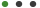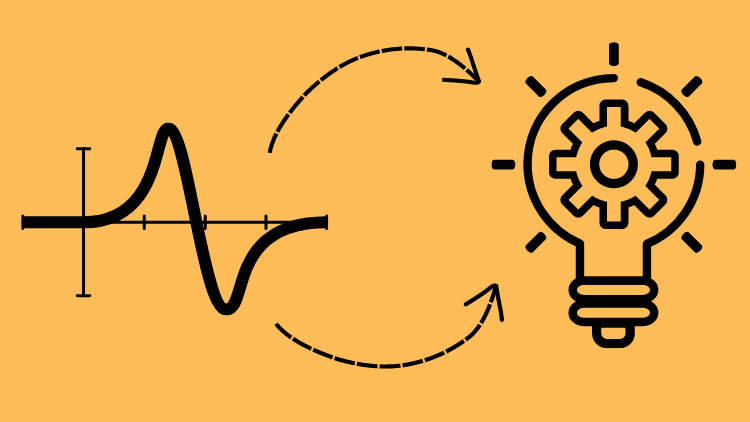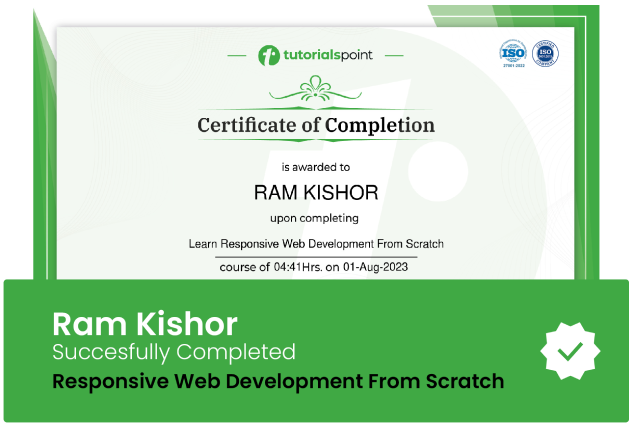### Calculus Masterclass: From Theory to Real World ApplicationsEmiliano Lako

# Calculus Masterclass: From Theory to Real World Applications

Unlocking the Power of Calculus: A Comprehensive Guide to Solving Complex Problems in Science, Engineering, and EconomicsUpdated on Sep, 2023Language - EnglishEmiliano LakoMath,Engineering,Calculus,Mathematics,Math Prep30-days Money-Back Guarantee

Training 5 or more people ?

## Course Description

Calculus is a fundamental branch of mathematics that has a wide range of applications across various fields, from natural sciences to engineering and economics. This masterclass provides a comprehensive introduction to calculus, covering its fundamental principles and real-world applications.

The masterclass will start with an overview of limits, derivatives, and integrals, which form the foundation of calculus. Through this, you will learn how to apply calculus to solve problems related to rates of change and optimization. Building on these concepts, the masterclass will delve into more advanced topics such as multivariable calculus, differential equations, and optimization techniques.

Multivariable calculus is a branch of calculus that deals with functions of more than one variable. Differential equations involve finding functions that satisfy specific conditions, and optimization techniques allow for the efficient and effective use of resources in various processes. You will learn how these topics apply to real-world problems and develop the necessary mathematical concepts, such as the chain rule and the fundamental theorem of calculus, to solve these problems.

The masterclass will also provide case studies from various fields to illustrate how calculus can be applied to solve practical problems. Through those examples, you will see firsthand how calculus can be applied to real-world situations and gain a deeper understanding of how to approach complex problems.

Whether you are a student seeking to improve your calculus skills or a professional looking to apply calculus to your work, this masterclass will equip you with a strong foundation in calculus theory and the practical skills necessary to apply it to real-world problems. By the end of this masterclass, you will have the knowledge and skills needed to solve complex problems in various fields using calculus.

### Goals

What will you learn in this course:

• Understand the fundamental principles of calculus, including limits, derivatives, and integrals
• Apply calculus to solve problems related to rates of change and optimization
• Develop an understanding of multivariable calculus and its applications
• Understand the basics of differential equations and their applications
• Learn optimization techniques and their application in various processes
• Apply calculus to real-world problems in various fields through case studies
• Develop a strong foundation in calculus theory and practical skills to solve complex problems in various fields using calculus

### Prerequisites

What are the prerequisites for this course?

• A solid understanding of algebra, trigonometry, and geometry
• A good understanding of functions, graphs, and equations
• A basic understanding of limits and derivatives
• Proficiency in solving mathematical problems and manipulating equations
• Familiarity with basic mathematical symbols and notations used in calculus.## Curriculum

Check out the detailed breakdown of what’s inside the course

Limits , Continuity, Differentiability
3 Lectures
•Limits (Algebraically) 07:55 07:55
•Visualize Limits Geometrically 04:00 04:00
•Continuity and Differentiability 10:29 10:29
Derivatives and their applications
3 LecturesIntegrals And Their Applications
4 LecturesSequences And Series
6 Lectures11 LecturesFourier Analysis And Laplace Transform
5 LecturesDifferential Equations
9 Lectures## Instructor DetailsEmiliano Lako

## Course Certificate

User your certification to make a career change or to advance in your current career. Salaries are among the highest in the world.